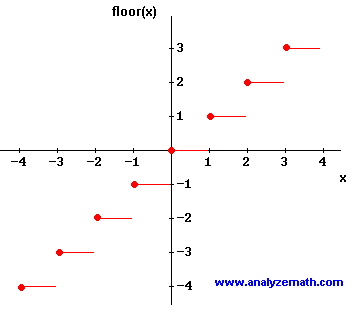# Floor Function

The floor function floor(x) is defined as the function that gives the highest integer less than or equal to x. The graph of floor(x) is shown below.The domain of floor(x) is the set of all real numbers, while the range of floor(x) is the set of all integers.

## Example

Evaluate floor(x) for various values of x.
floor(1.5) = 1
floor(0) = 0
floor(2) = 2
floor(- 3) = - 3
floor(- 1.5) = - 2